# NCERT Exemplar Class 11 Maths Solutions for Chapter 8 - Binomial Theorem

NCERT Exemplar Class 11 Maths Chapter 8 Binomial Theorem, are provided here for students to learn and prepare for the exam. Learn all the concepts related to this chapter of Binomial theorem with the help of NCERT exemplars prepared by our experts and in accordance with CBSE syllabus (2018-2019). Students of class 11 can download the pdf format of these materials and practice it offline as well.

## Class 11 Maths NCERT Exemplar Problems on Binomial Theorem

In chapter 8 exemplars, students will learn to solve problems based on the topics given below;

• Binomial Theorem for Positive Integral Indices
• Pascal’s Triangle
• Binomial theorem  for any positive integer n
• General and Middle Terms for Binomial expansion

A Polynomial with two terms is termed as a Binomial and the theorem which describes the algebraic expansion of powers of a binomial is the Binomial Theorem. It is one of the quintessential theorems which is used to expand polynomials into a sum, where the exponents are nonnegative integers along with the coefficient of each term, as a positive integer.

The Binomial Coefficients are the coefficients that appear in the binomial expansion. They can be represented as:

1. Combinatorial Interpretation
2. Formulae

Also, while preparing for final exams they can use it as a reference tool to clarify their doubts and resolve the difficulties faced during solving the problems.

Apart from these exemplars, students are also suggested to solve previous year question papers and sample papers to know the question pattern and marking scheme for chapter 8, Binomial Theorem. Also, students can get other learning materials such as NCERT solutions, notes and question papers online for free, to learn and prepare for the class 11th exam.

The NCERT Exemplar for Class 11 Maths Chapter 8 Binomial Theorem PDF can be downloaded below: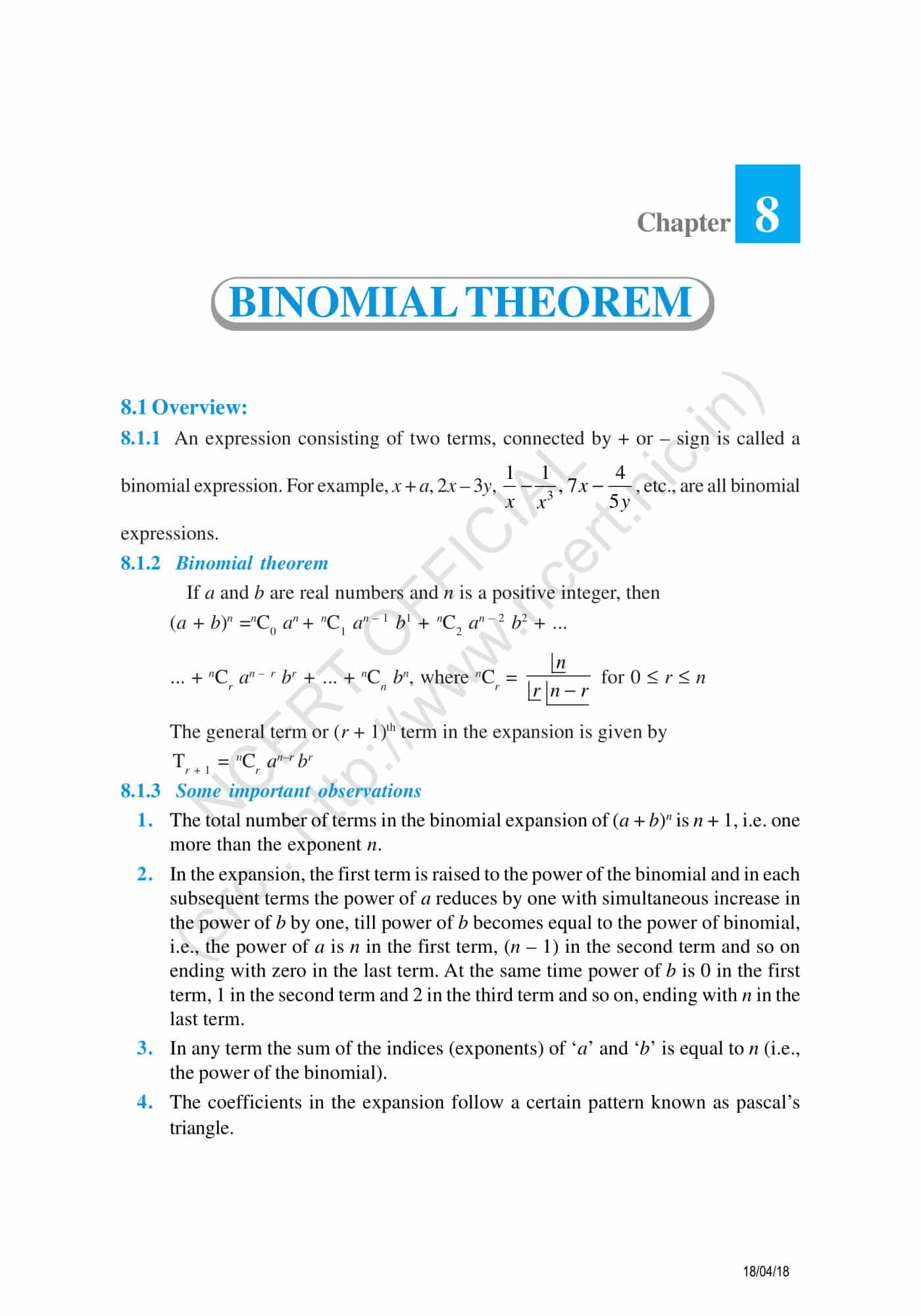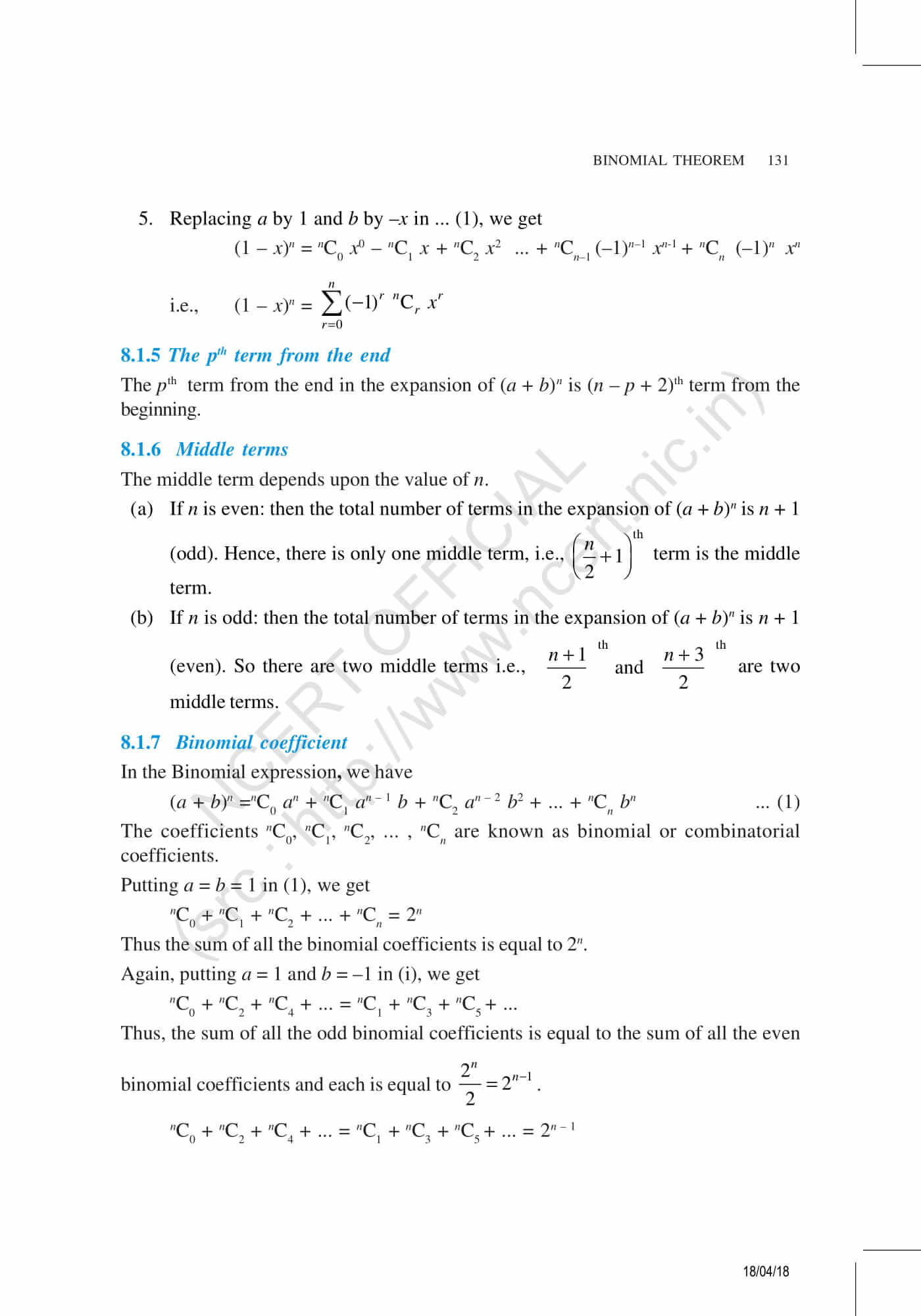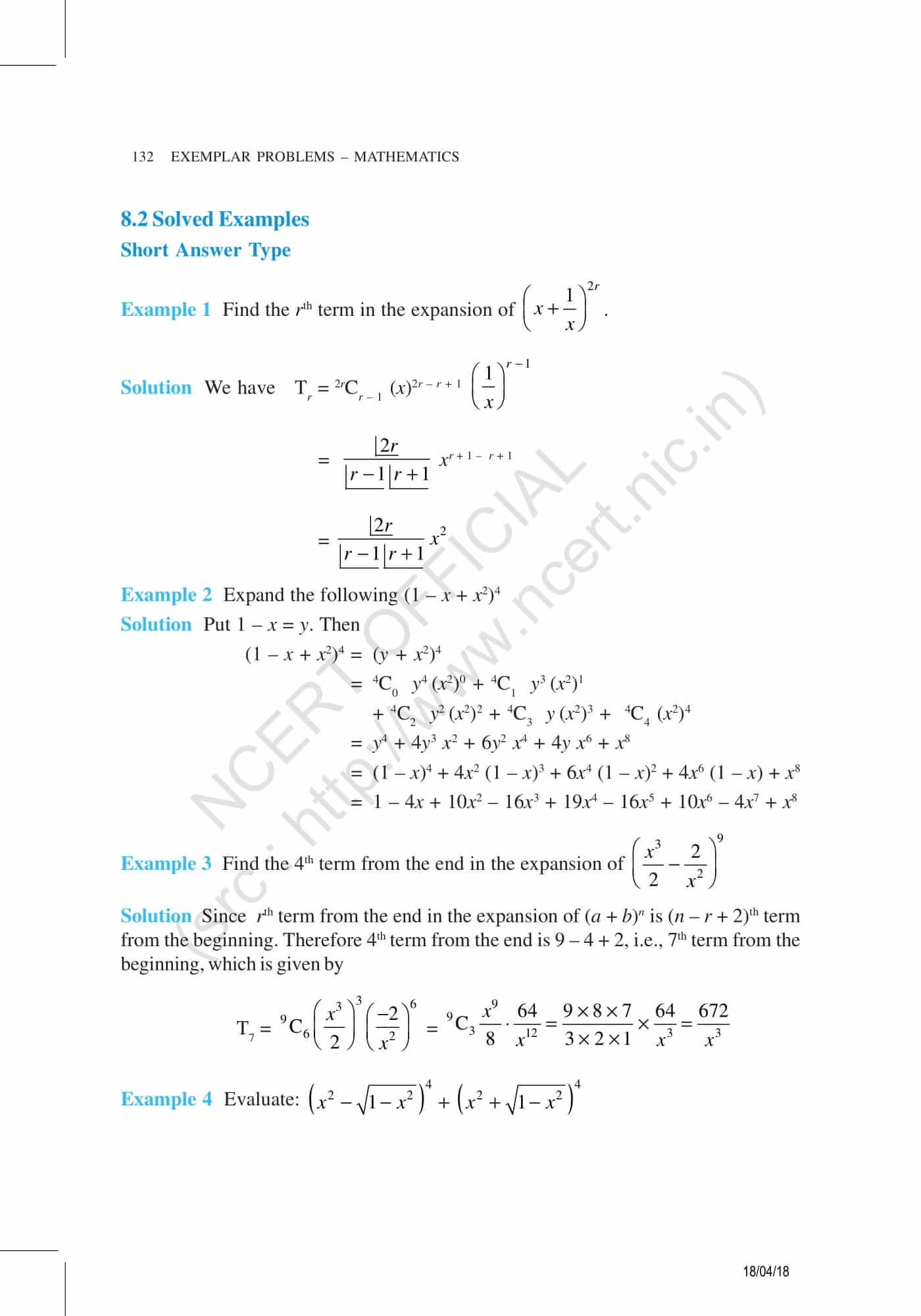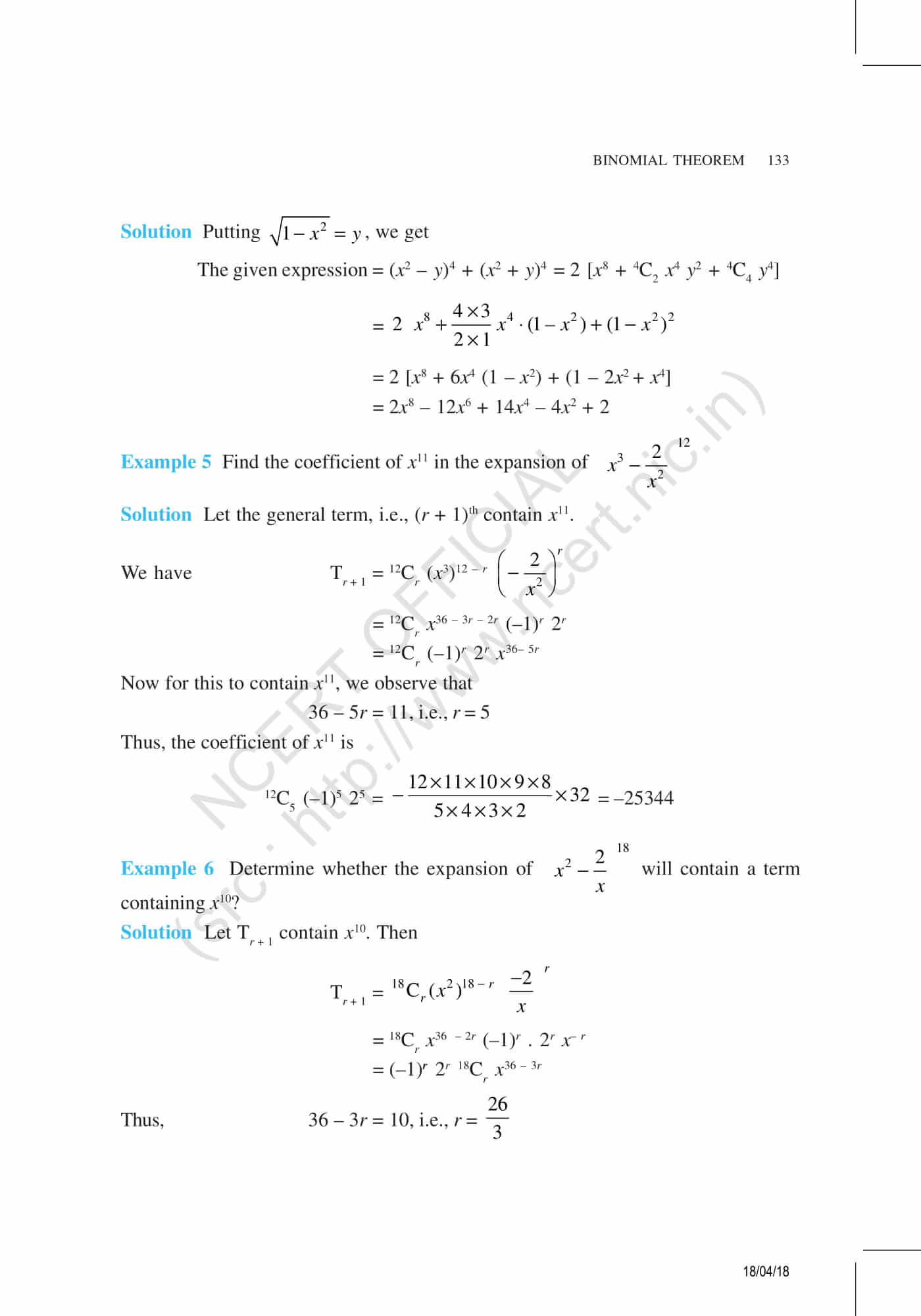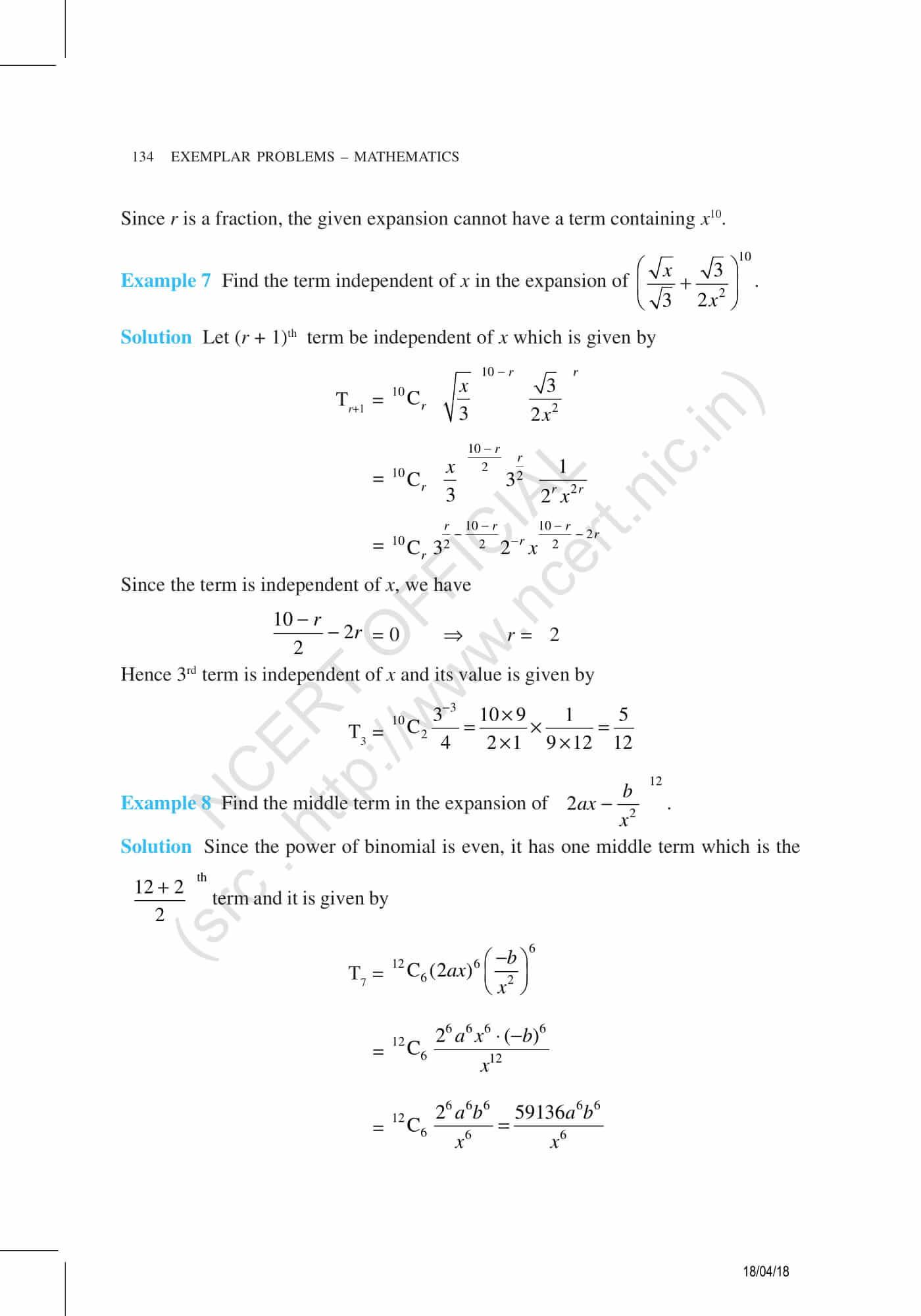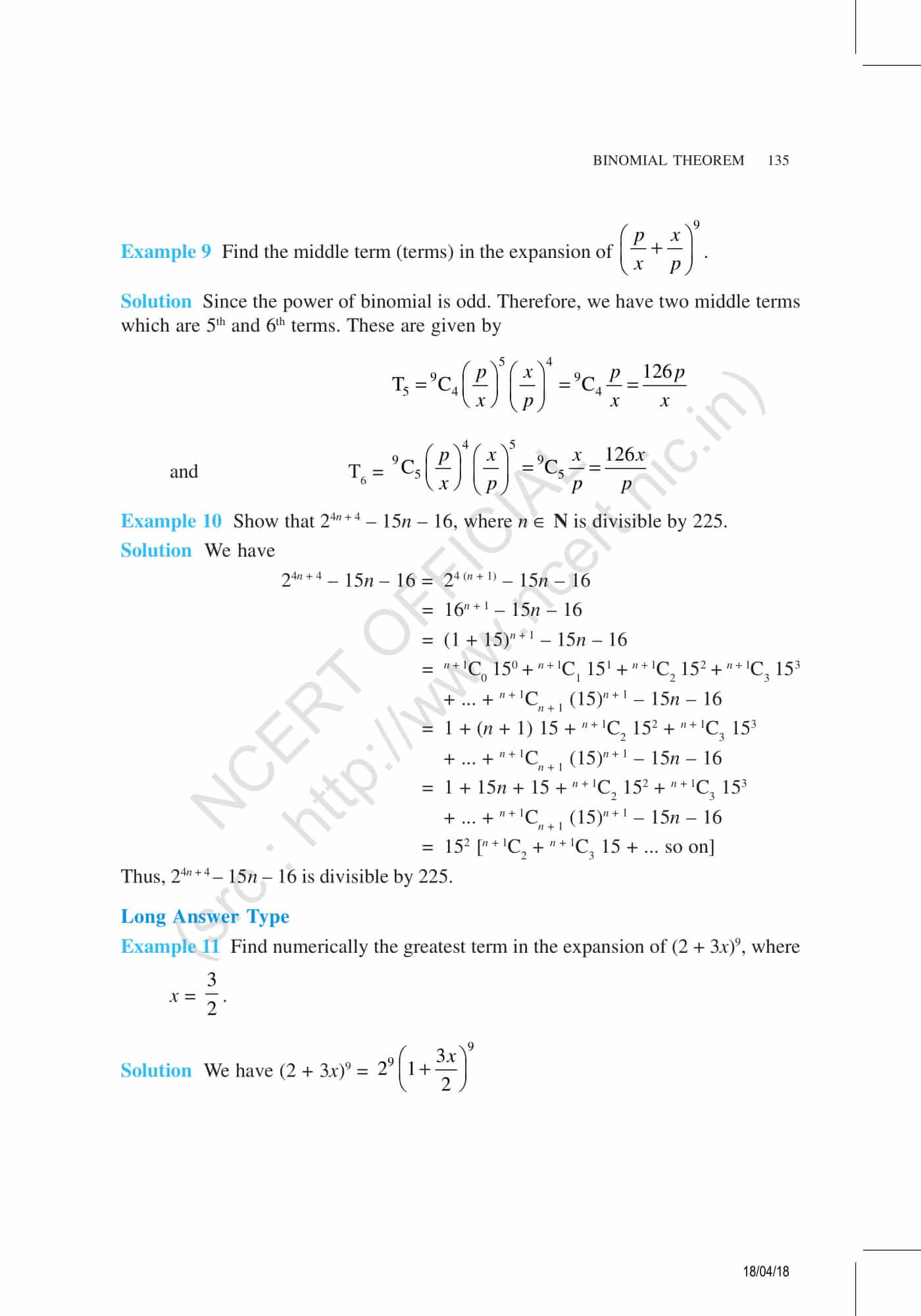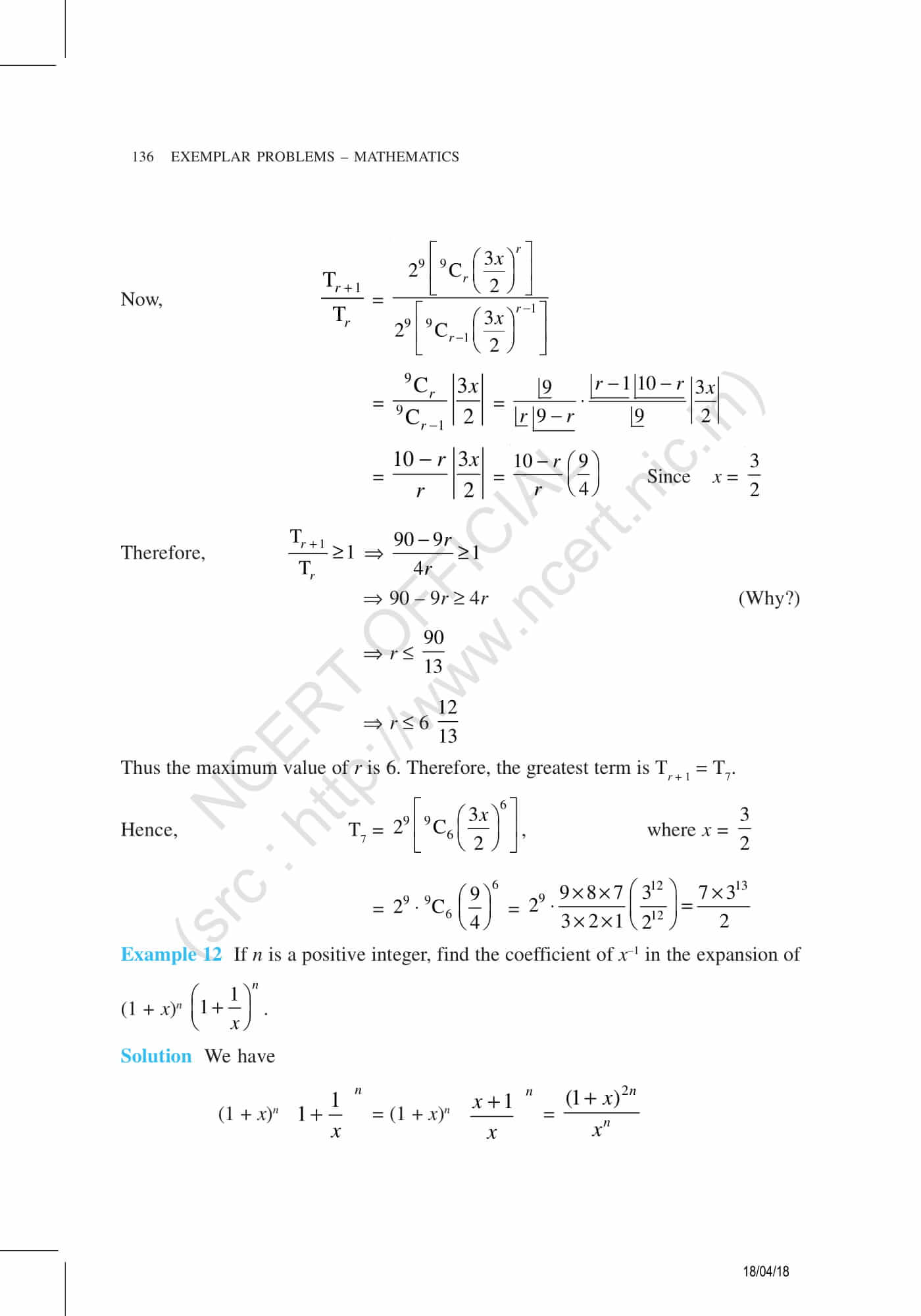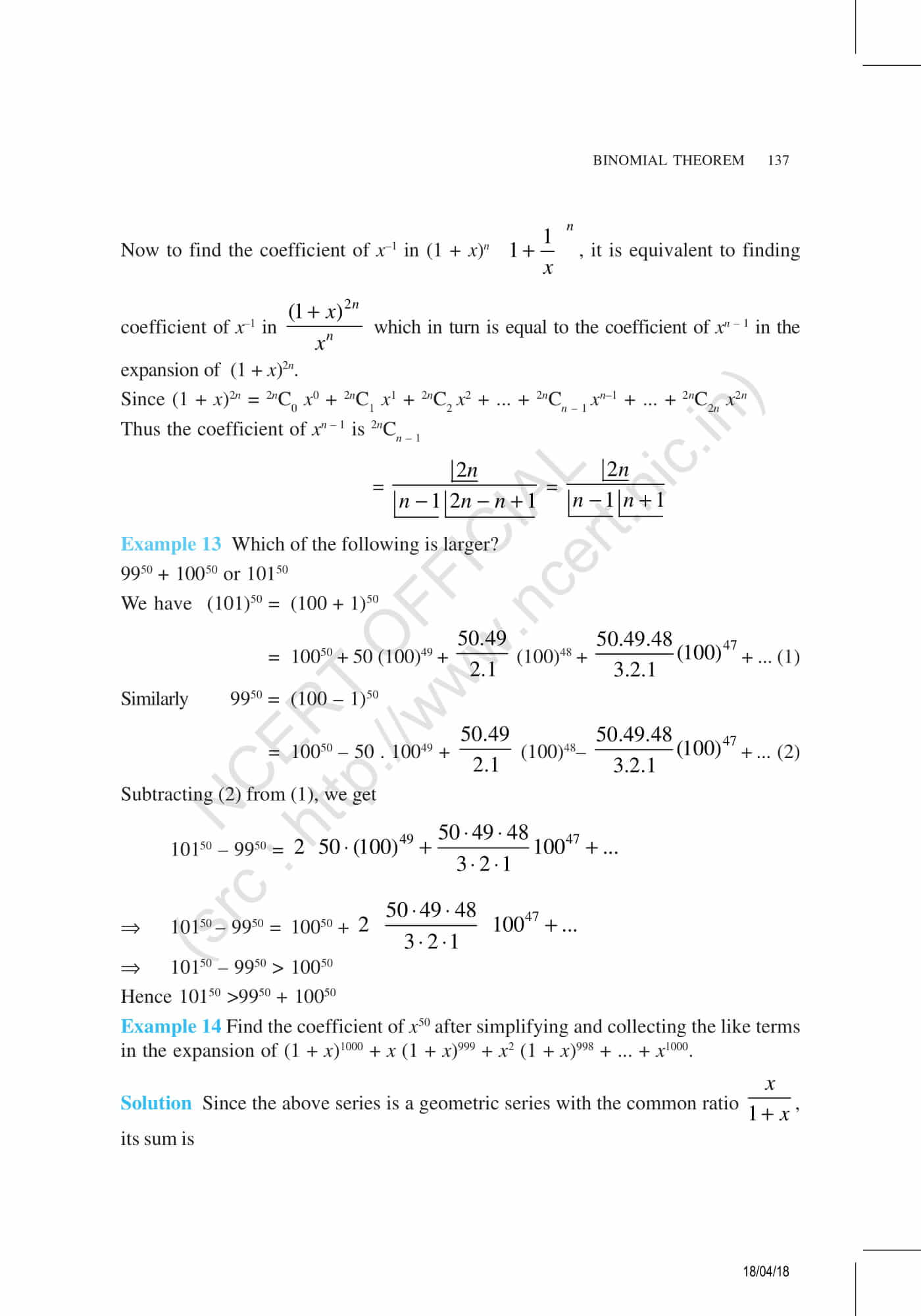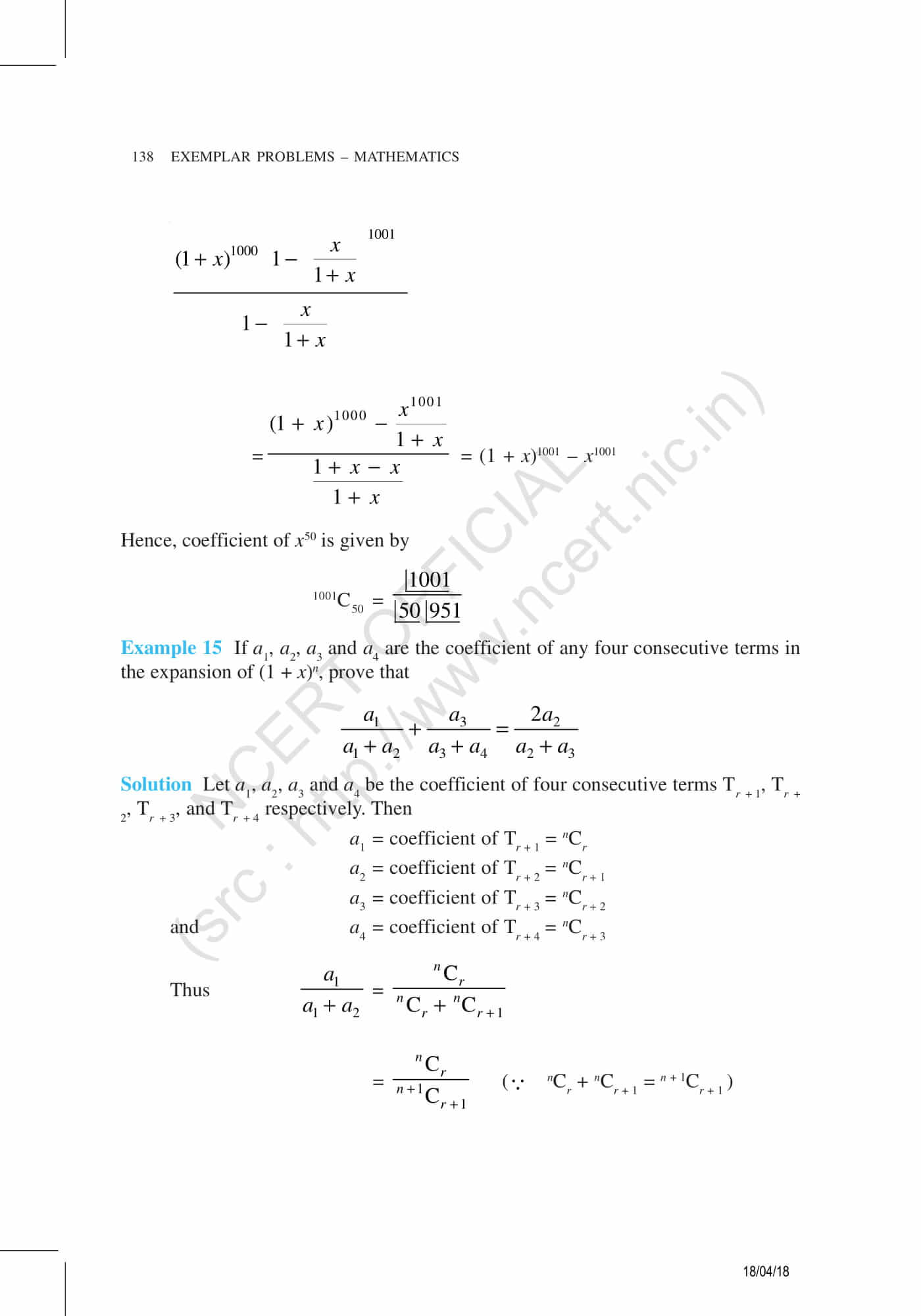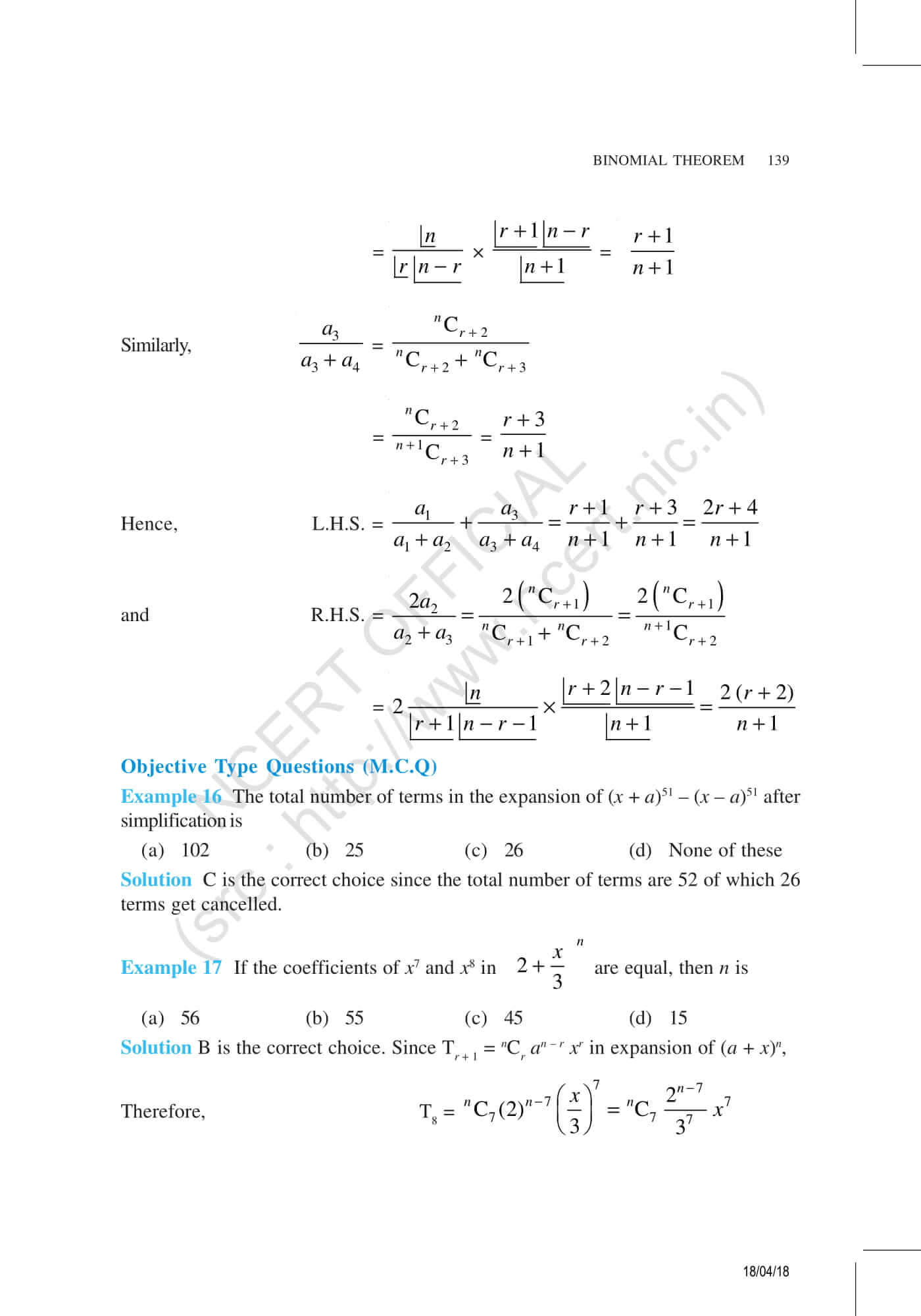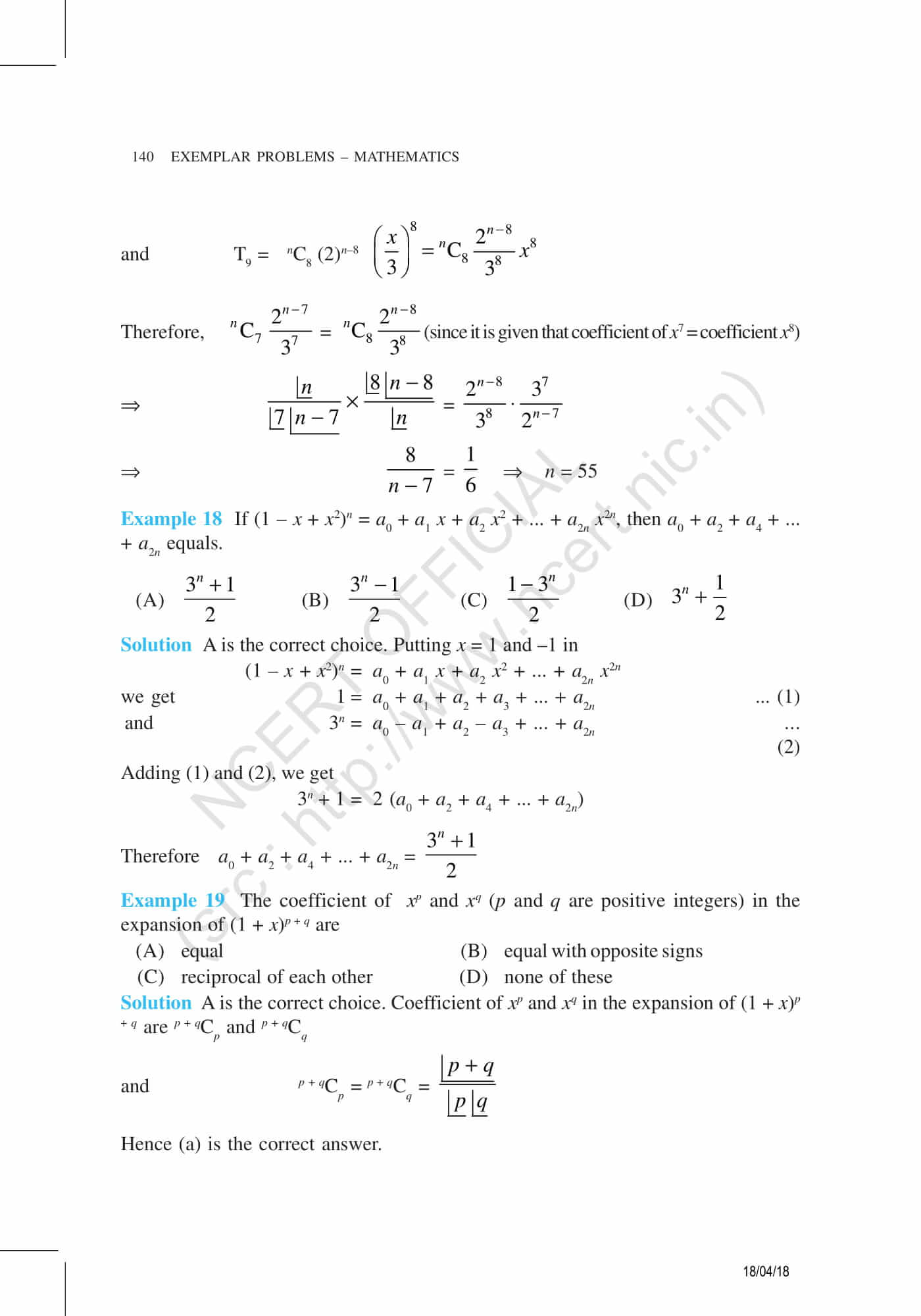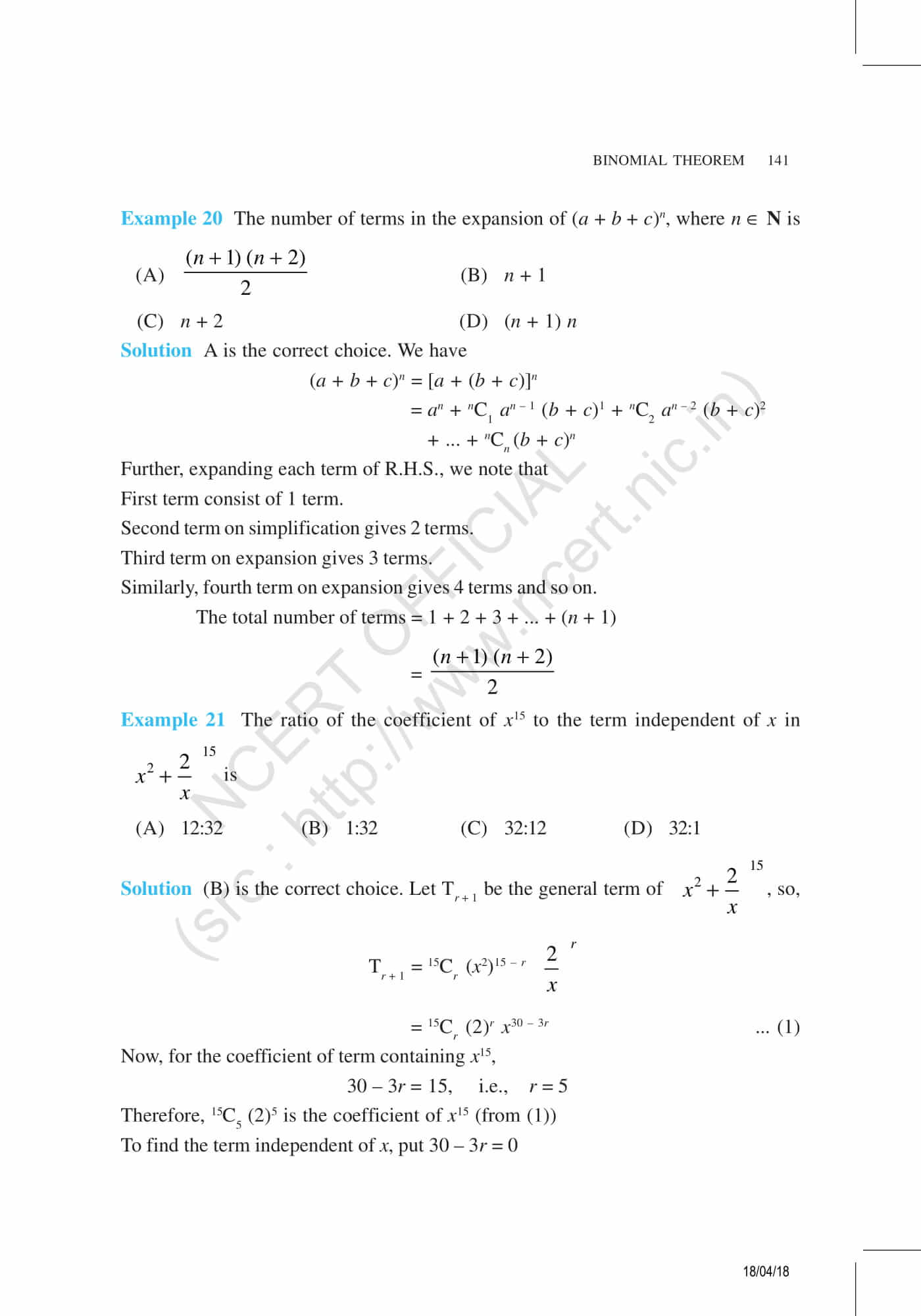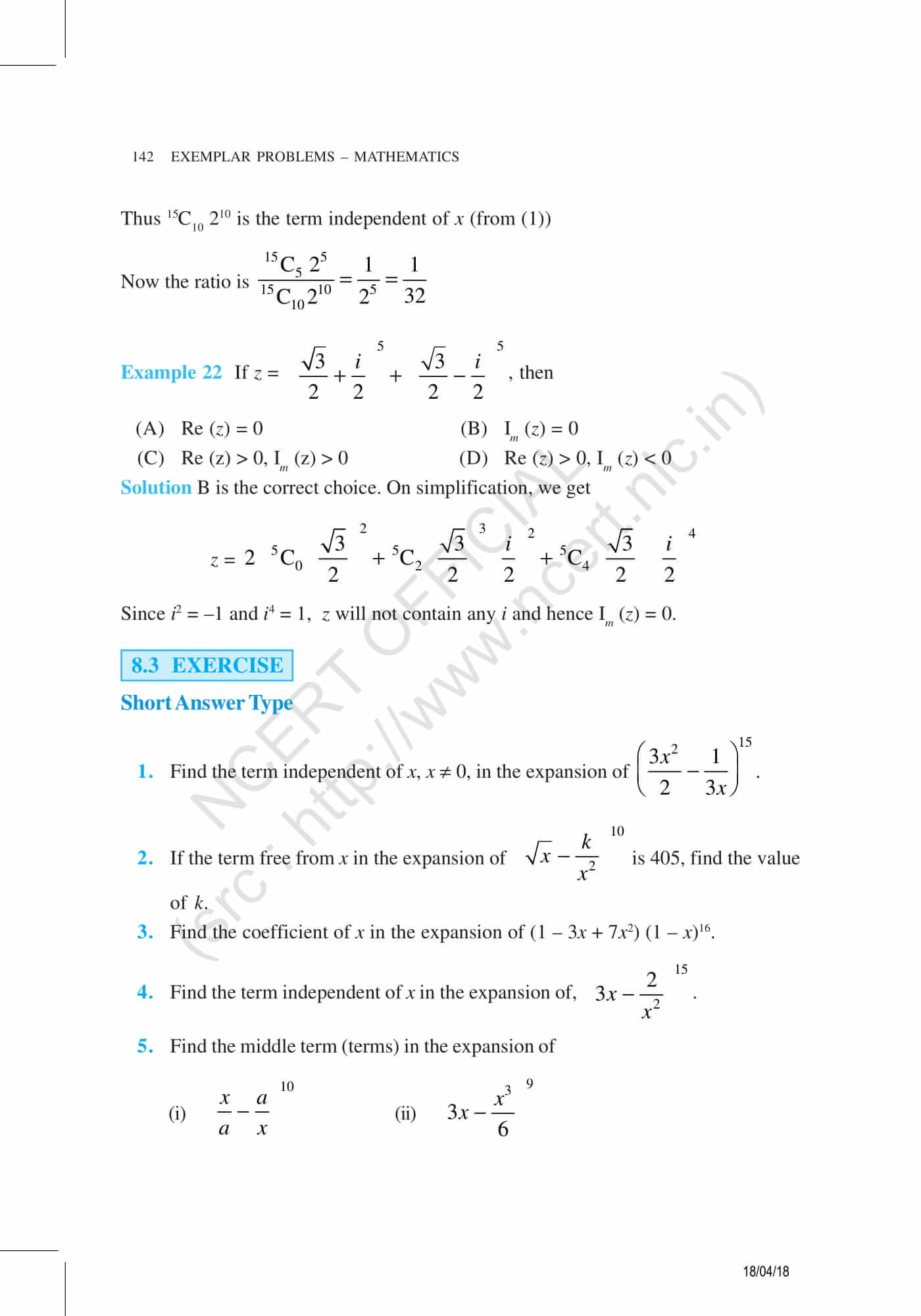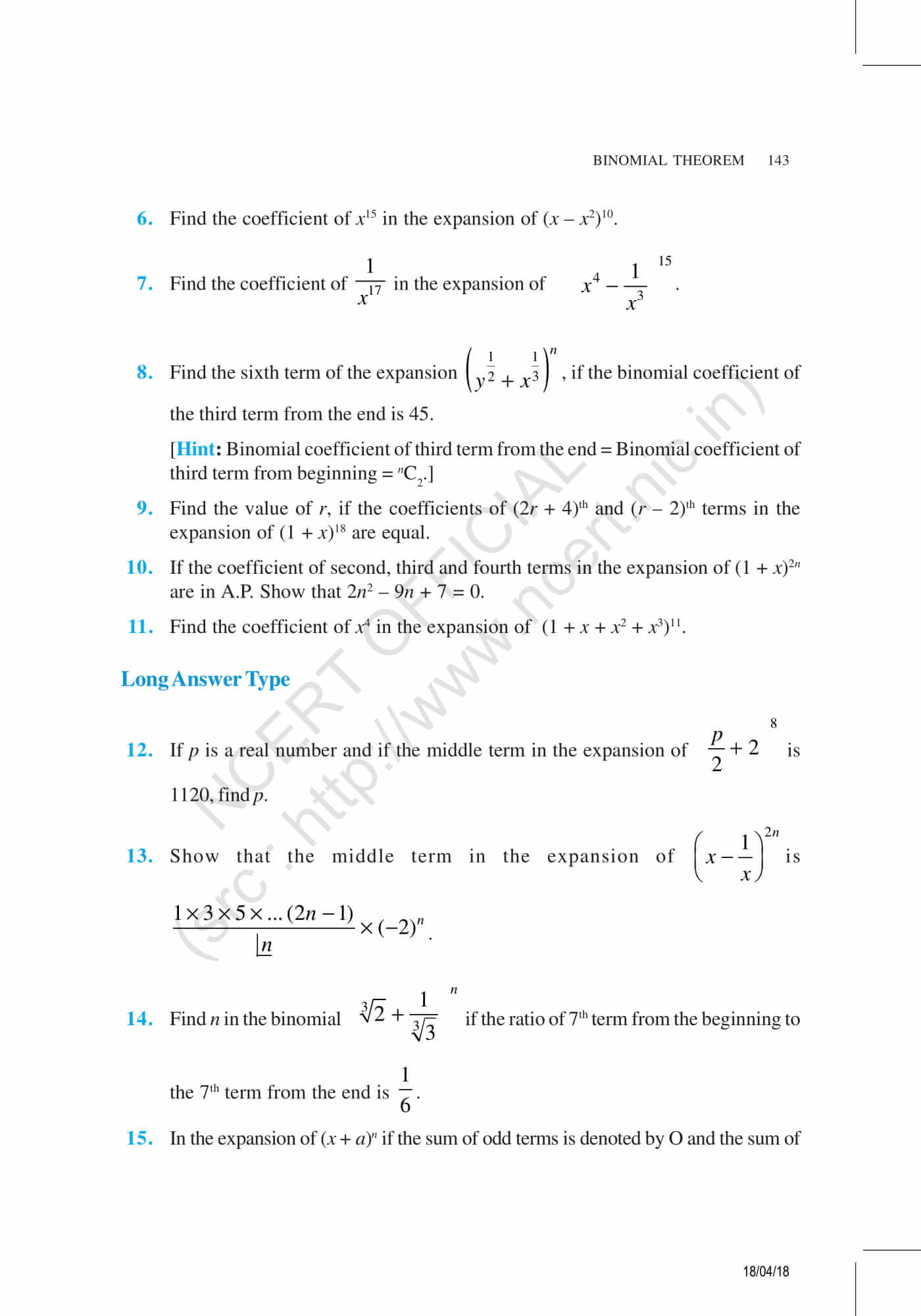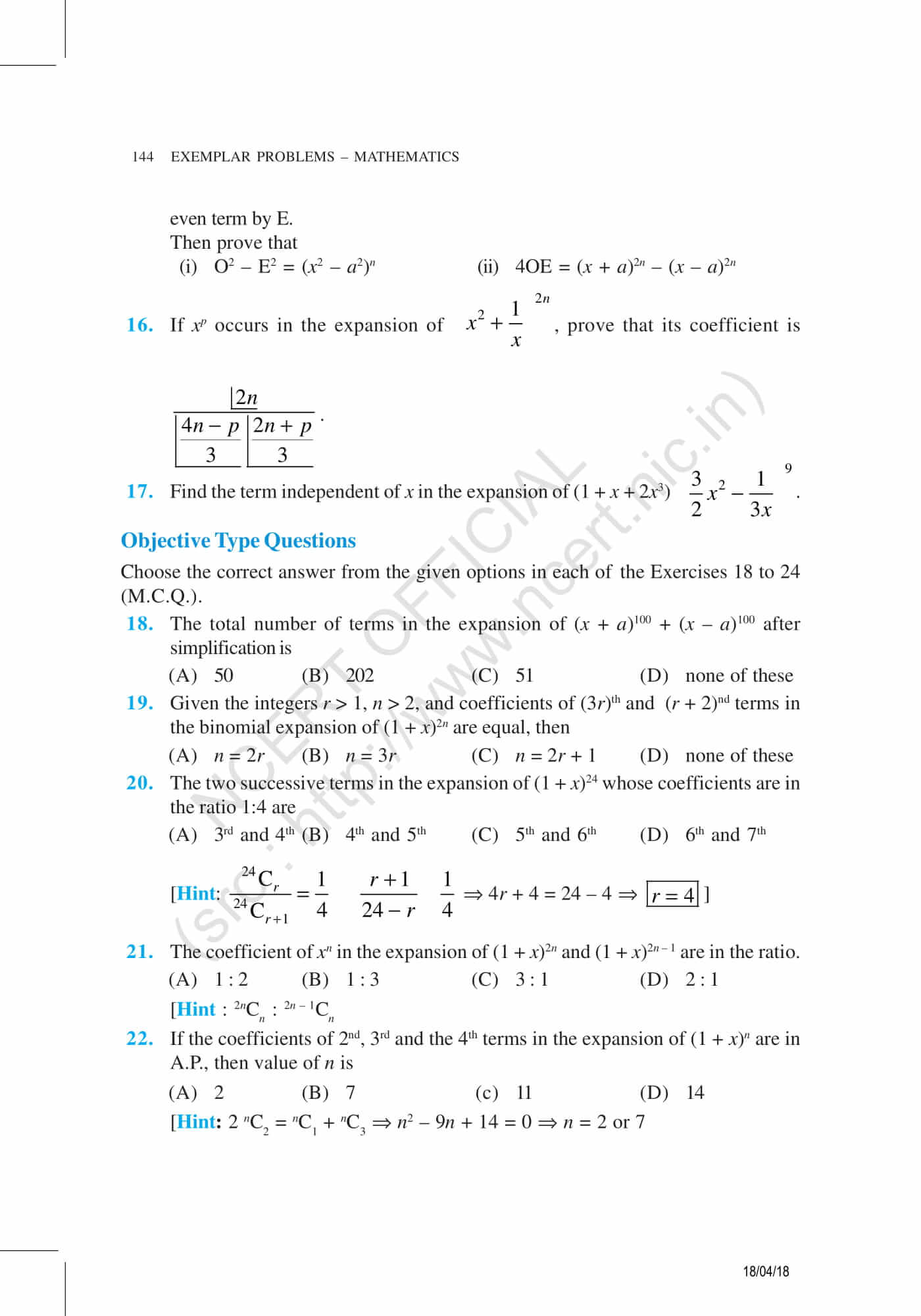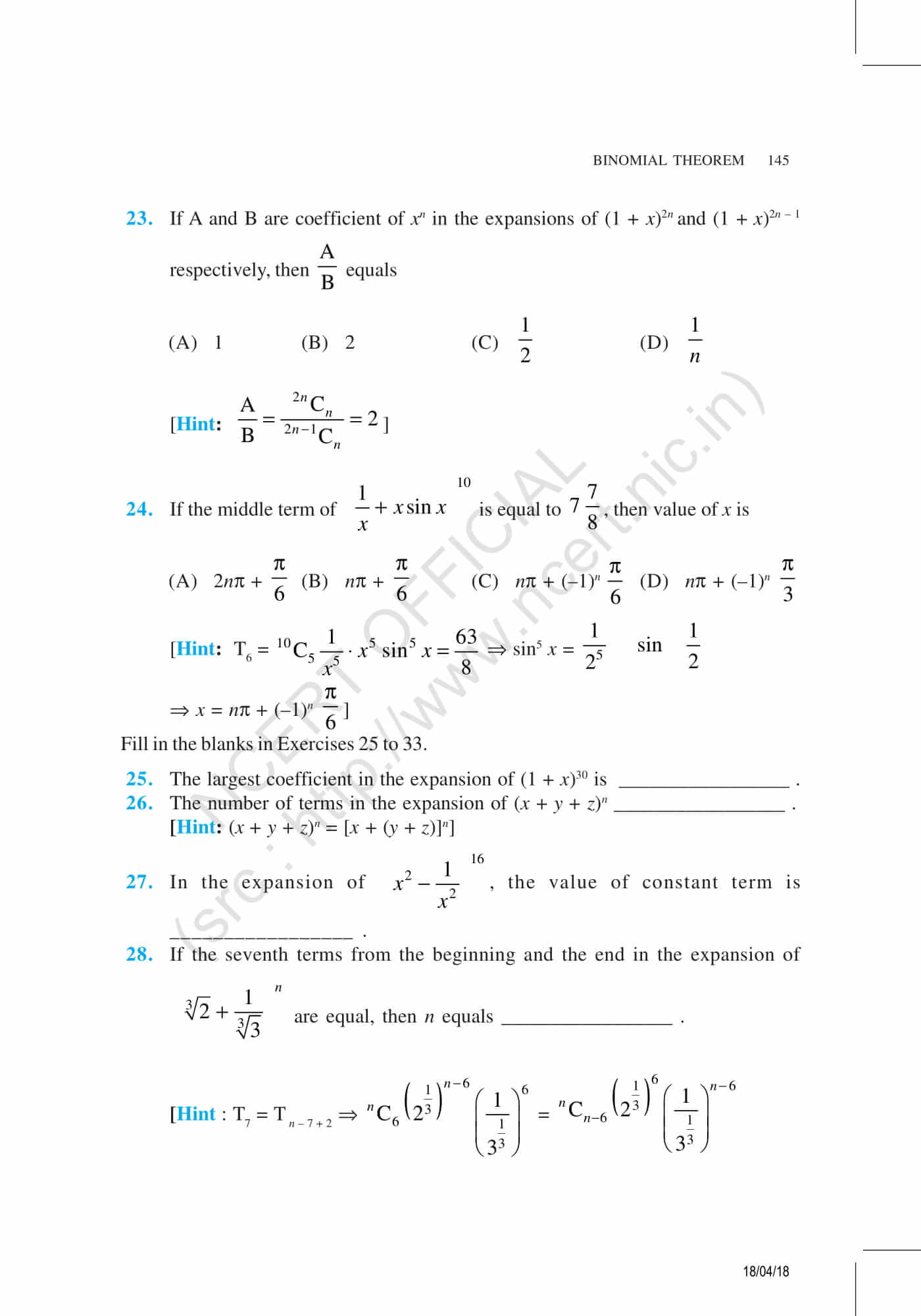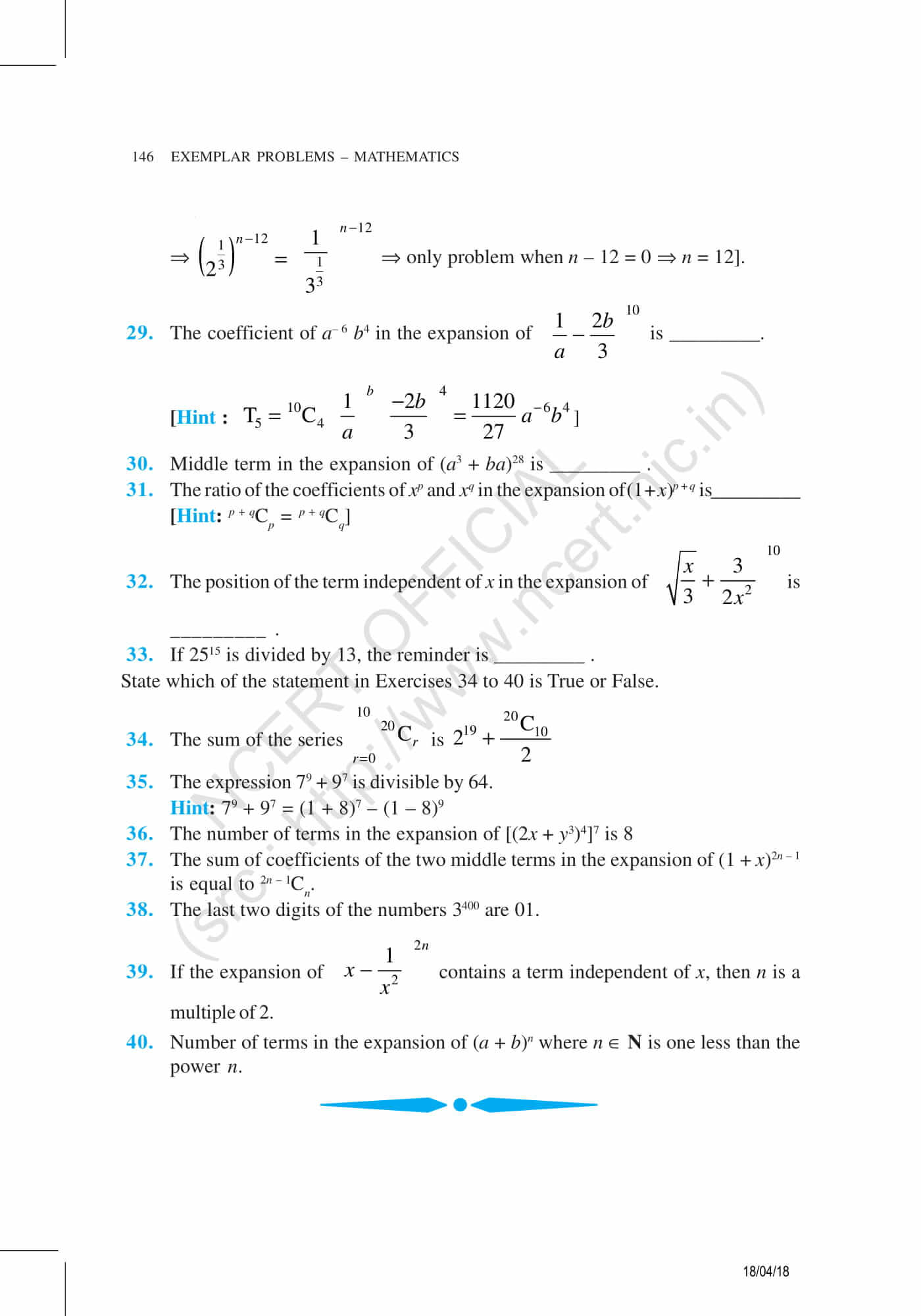BYJU’S provides exemplar problems and online learning materials for all the classes to make them score well in exams. Enjoy with the help of educational and interactive videos, by downloading BYJU’S- The Learning App and get a better learning experience.